# Primitive recursion

(diff) ← Older revision | Latest revision (diff) | Newer revision → (diff)

A means of defining functions with natural number arguments and values. One says that an-place function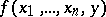is obtained by primitive recursion from an-place functionand an-place function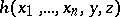if for all natural number values ofone hasand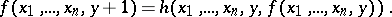For givenandsuch a functionexists always and is unique. For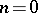the defining equations forcan be written as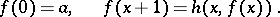A fundamental property of primitive recursion is that for any meaningful specification of the notion of computability, a functionobtained from computable functionsandby means of primitive recursion is itself computable (cf. Computable function). Primitive recursion is one of the basic ways for generating all primitive recursive and all partial recursive functions from an initial set of basic functions (cf. Primitive recursive function; Partial recursive function).

How to Cite This Entry:
Primitive recursion. Encyclopedia of Mathematics. URL: http://encyclopediaofmath.org/index.php?title=Primitive_recursion&oldid=17879
This article was adapted from an original article by S.N. Artemov (originator), which appeared in Encyclopedia of Mathematics - ISBN 1402006098. See original article